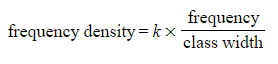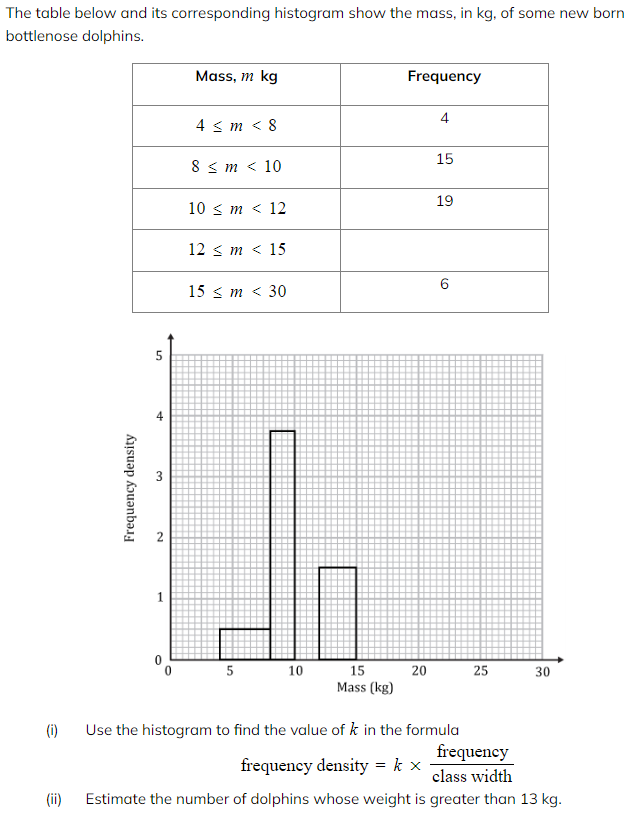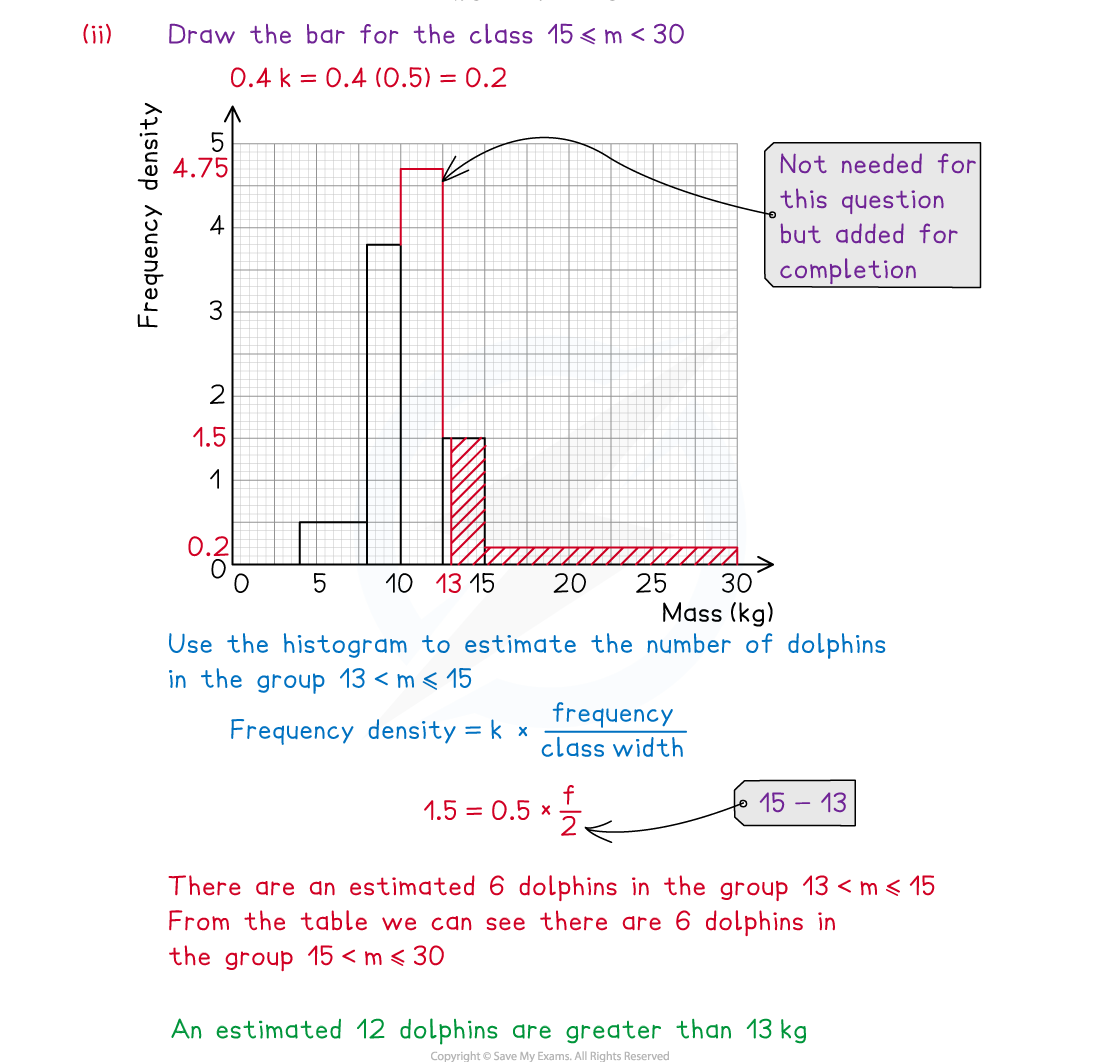# AQA A Level Maths: Statistics复习笔记2.2.3 Histograms

### Histograms

#### What is a histogram?

• A histogram is similar to a bar chart but with some key differences
• A histogram is for displaying grouped continuous data whereas a bar chart is for discrete or qualitative data
• There will never be any gaps between the bars of adjacent groups in a histogram
• Whilst in a bar chart the frequency is read from the height of the bar, in a histogram the height of the bar is the frequency density
• On a histogram frequency density is plotted on the y – axis
• This allows a histogram to be plotted for unequal class intervals
• It is particularly useful if data is spread out at either or both ends
• The area of each bar on a histogram will be proportional to the frequency in that class

#### What are the key features of a histogram?

• You will not be asked to draw a histogram but you may have to add information to one so you should make sure you are familiar with the process for drawing one
• Step 1.  Always check that there are no gaps between the upper boundary of a class and the    lower boundary of the next class
• If there are gaps you will need to close them by changing the boundaries before carrying out any calculations
• Consider whether the values are rounded or truncated before closing the gaps
• Step 2.  Find the class width of each group by subtracting the lower boundary from the upper boundary
• Step 3.  Calculate the frequency density for each group using the formula:• Step 4.  The histogram will be drawn with the data values on the x – axis and frequency density on the y – axis
• Remember that the scale on both axes must be even, although the class widths may be uneven
• Both axes should be clearly labelled and units included on the x – axis
• Most often, the bars will have different widths
• Occasionally you will be asked to add a frequency polygon to the histogram
• This is done by joining up the midpoints at the top of each bar
• You should not join up the first or last midpoint to the x – axis (it is not really a polygon!)

#### How do we interpret a histogram?

• It is important to remember that the y – axis does not tell us the frequency of each bar in the histogram
• The area of the bar gives information about the frequency
• Most of the time, the frequency will be the area of the bar and is found by multiplying the class width by the frequency density
• Occasionally, the frequency will be proportional to the area of the bar
•In these cases more information will be given to help you find the value of k
• You may be asked to find the frequency of part of a bar within a histogram
• Find the area of that section of the bar using any information you have already found out
• You will need to have found the value of k first

#### Worked Example#### Exam Tip

• Remember that the area of a bar in a histogram is not always the frequency itself but could be proportional to the frequency. Look carefully at the scales on the axes, it will rarely be a simple 1 unit to 1 square.